Question

Problem 05 In R Out For the circuit above, answer the following questions. 1. Derive the formula ...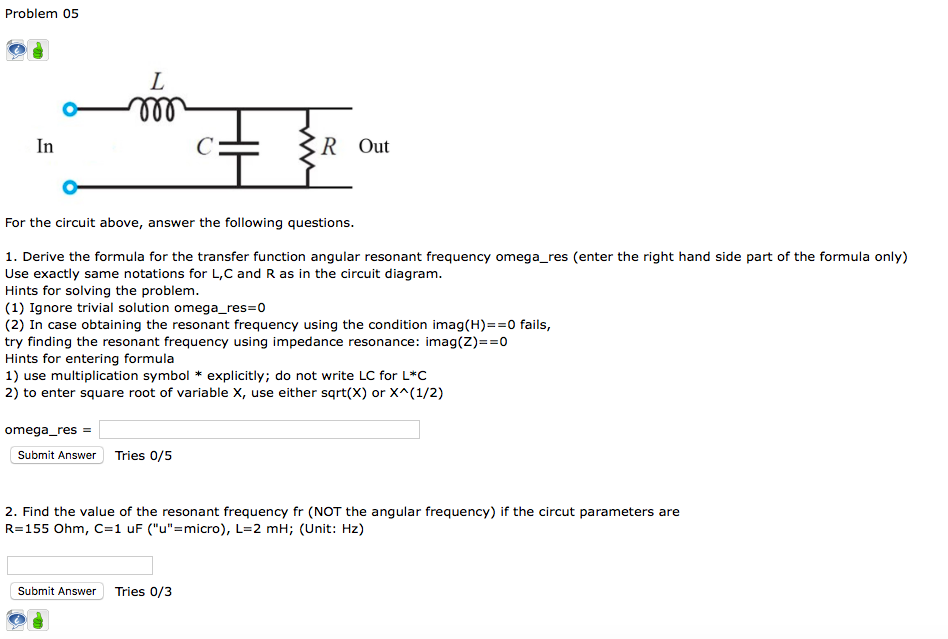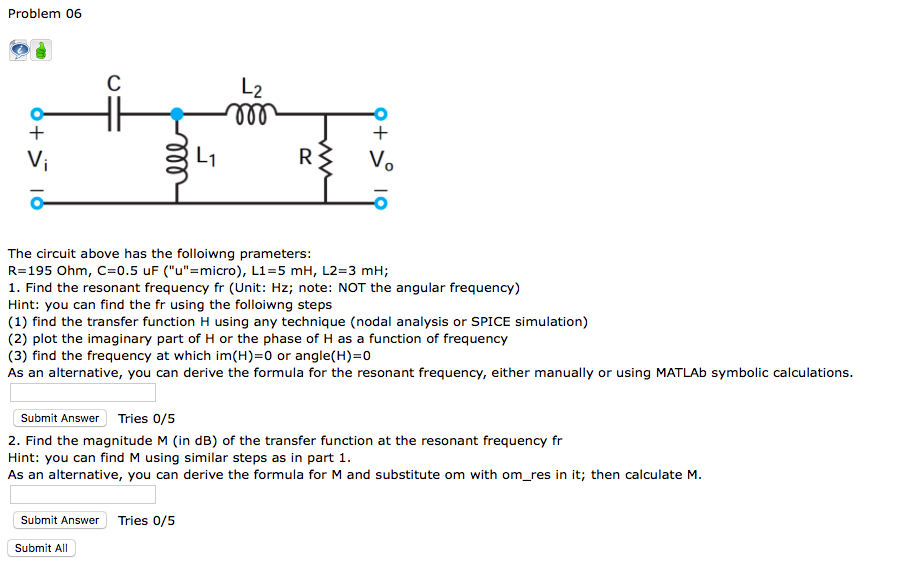Problem 05 In R Out For the circuit above, answer the following questions. 1. Derive the formula for the transfer function angular resonant frequency omega_res (enter the right hand side part of the formula only) Use exactly same notations for L,C and R as in the circuit diagram. Hints for solving the problem. (1) Ignore trivial solution omega_res 0 (2) In case obtaining the resonant frequency using the condition imag(H)0 fails, try finding the resonant frequency using impedance resonance: imag(Z)0 Hints for entering formula 1) use multiplication symbol * explicitly; do not write LC for L*C 2) to enter square root of variable X, use either sqrt(X) or X(1/2) omega-res = Submit Answer Tries 0/5 2. Find the value of the resonant frequency fr (NOT the angular frequency) if the circut parameters are R=155 Ohm, C=1 uF ("u"-micro), L= 2 mH; (Unit: Hz) Submit Answer Tries 0/3
Problem 06 し2 R3 V The circuit above has the folloiwng prameters: R= 195 Ohm, C=0.5 uF ("u"-micro), L1 = 5 mH, L2-3 mH; 1. Find the resonant frequency fr (Unit: Hz; note: NOT the angular frequency) Hint: you can find the fr using the folloiwng steps (1) find the transfer function H using any technique (nodal analysis or SPICE simulation) (2) plot the imaginary part of H or the phase of H as a function of frequency (3) find the frequency at which im(H) 0 or angle(H)-0 As an alternative, you can derive the formula for the resonant frequency, either manually or using MATLAb symbolic calculations Submit Answer Tries 0/5 2. Find the magnitude M (in dB) of the transfer function at the resonant frequency fr Hint: you can find M using similar steps as in part 1. As an alternative, you can derive the formula for M and substitute om with om_res in it; then calculate M Submit Answer Tries 0/5 Submit All

As per HomeworkLib policy we need to answer only the first question if multiple questions are posted.... Please upload remaining questions separately... Please find the explanation below for 1st question.... Hope you understand... Thanks in advance....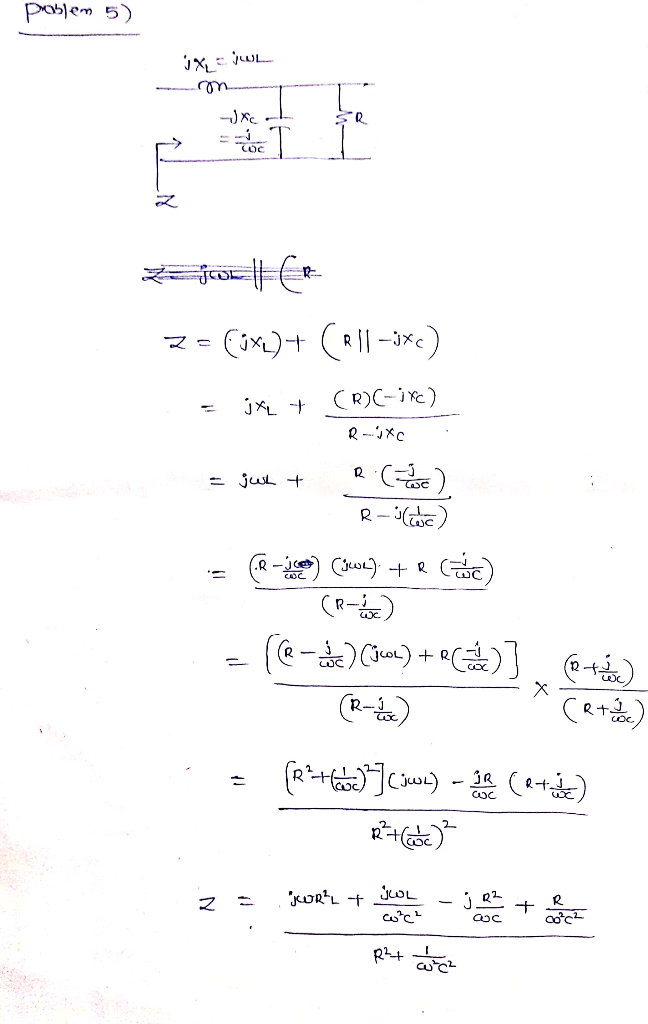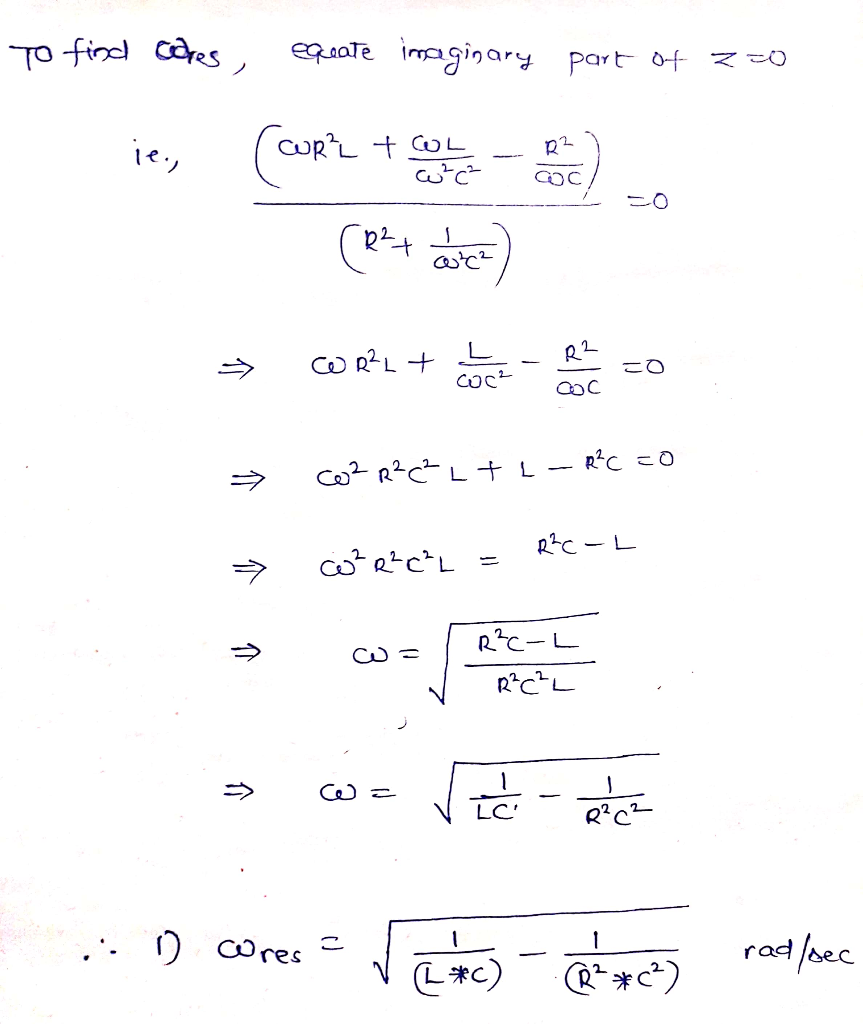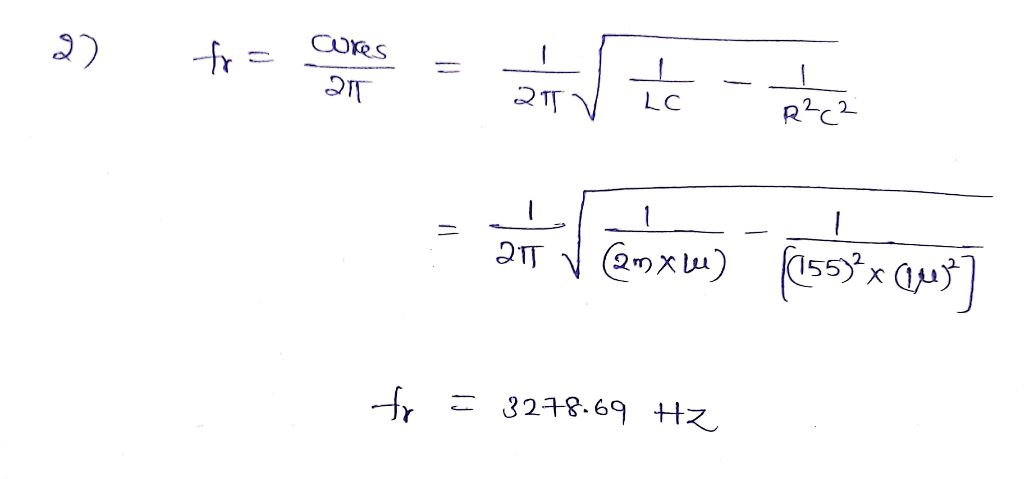Earn Coins

Coins can be redeemed for fabulous gifts.

Similar Homework Help Questions
• The circuit above has the following parameters: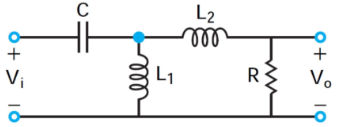The circuit above has the following parameters: R=55 Ohm, C=1.5 uF ("u"=micro), L1=3 mH, L2=4 mH; Using MATLAB (or equivalent tool) generate the transfer function H = Vo/Vi magnitude MdB(f) and Phase(f) frequency dependencies in the frequency range from 100 Hz to 12000 Hz. Define the Magntude plot of the transfer function by entering f (Hz) and M (dB) points. Do not enter units.Define the Phase plot of the transfer function by entering f (Hz) and Phase (in degrees) points. Do not enter...

• Input voltage is applied to a circuit consisting of a series connection of capacitor C=10 uF...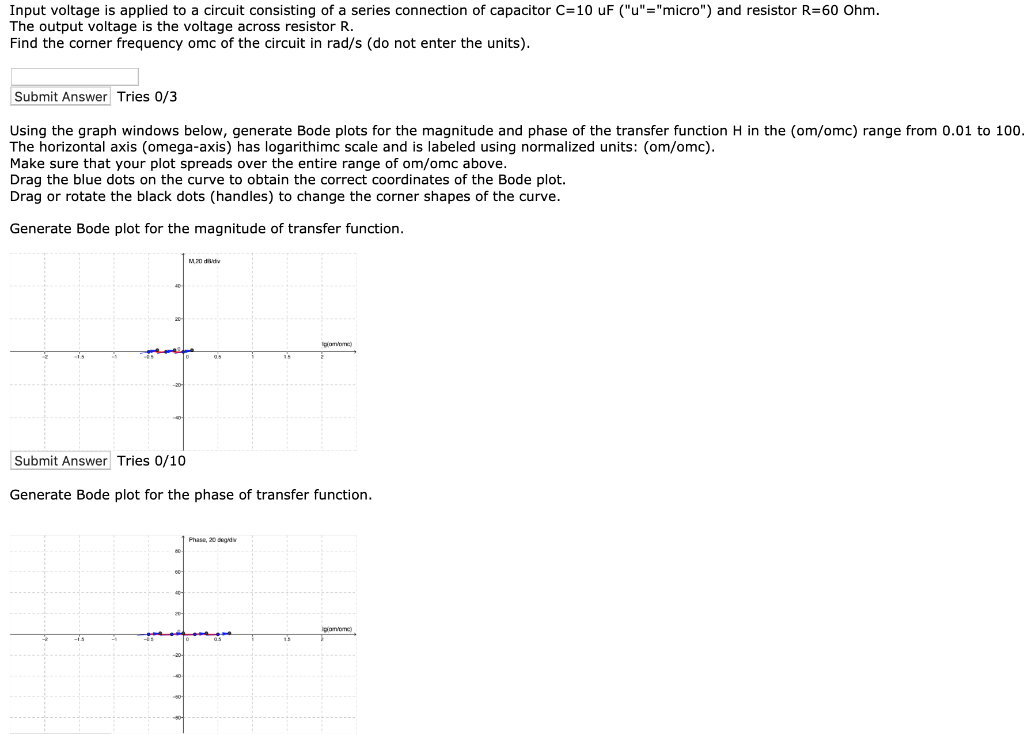Input voltage is applied to a circuit consisting of a series connection of capacitor C=10 uF ("u"="micro") and resistor R=60 Ohm. The output voltage is the voltage across resistor R. Find the corner frequency omc of the circuit in rad/s (do not enter the units). Submit Answer Tries 0/3 Using the graph windows below, generate Bode plots for the magnitude and phase of the transfer function H in the com/omc) range from 0.01 to 100- The horizontal axis (omega-axis) has...

• Problem #17 In the following circuit,L2-5.6 mH, R-5 Ohm, C-8.6uF, angular frequency w 10000 rad/s. The...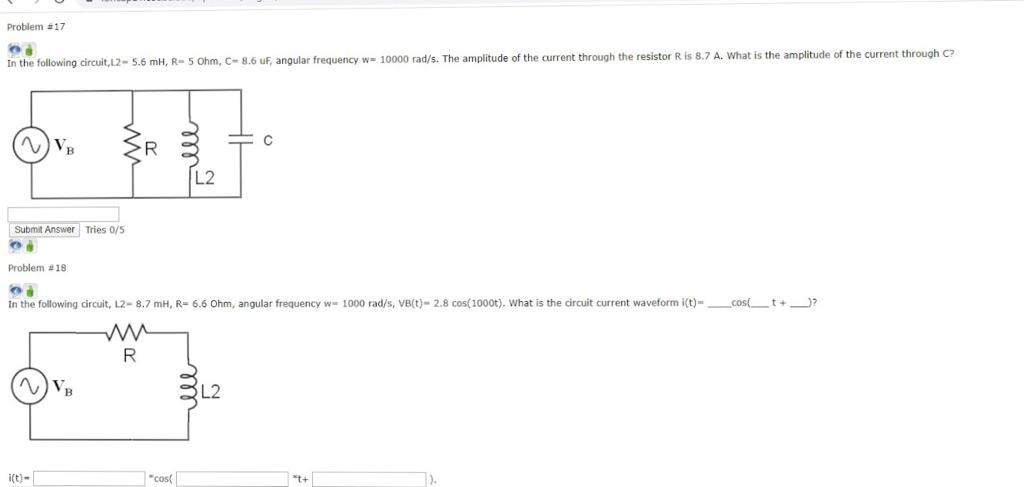Problem #17 In the following circuit,L2-5.6 mH, R-5 Ohm, C-8.6uF, angular frequency w 10000 rad/s. The amplitude of the current through the resistor Ris 8.7 A. What is the amplitude of the current through C? [ 12 Submit Answer Tries 0/5 Problem #18 In the following circuit, 12- 8.7 mH, R-6.6 Ohm, angular frequency w 1000 rad/s, VB(t)- 2.8 cos(1000t). What is the circuit current waveform (t) _ cos_ t+ )? R ve ZL2 cos

• Matlab Code if available Problem 04 mm us(0 In the circuit, the voltage source Vs(t) frequency,...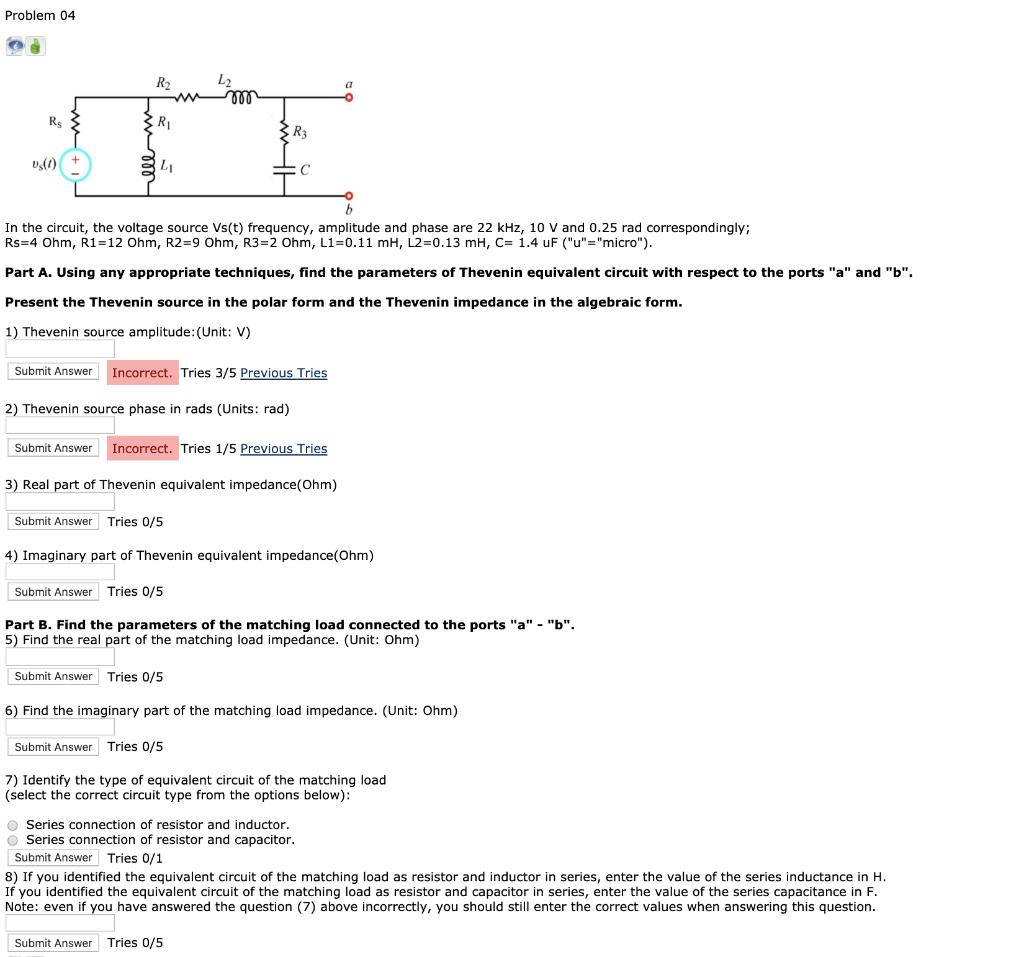Matlab Code if available Problem 04 mm us(0 In the circuit, the voltage source Vs(t) frequency, amplitude and phase are 22 kHz, 10 V and 0.25 rad correspondingly; Rs=4 Ohm, Ri=12 Ohm, R2=9 Ohm, R3=2 Ohm, L1=0.11 mH, L2=0.13 mH, C= 1.4 uF ("u"="micro"). Part A. Using any appropriate techniques, find the parameters of Thevenin equivalent circuit with respect to the ports "a" and "b". Present the Thevenin source in the polar form and the Thevenin impedance in the algebraic...

• Problem #6 m Di ZR S T In the following circuit, L1= 5 mH, L2= 2...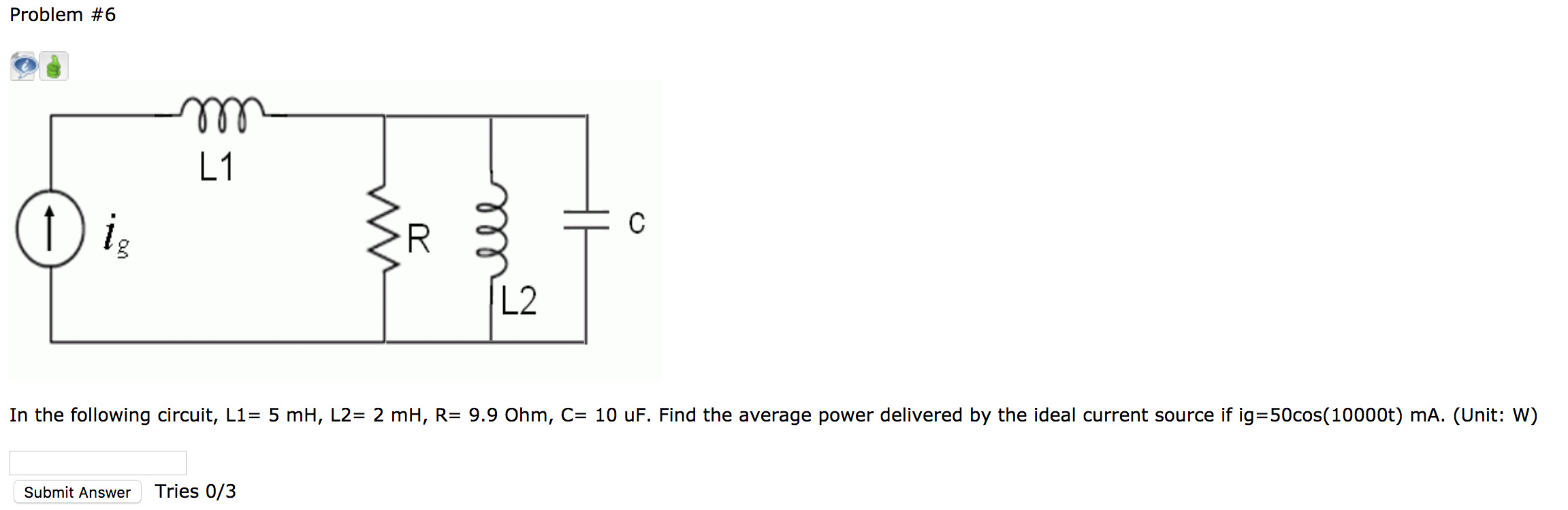Problem #6 m Di ZR S T In the following circuit, L1= 5 mH, L2= 2 mH, R= 9.9 Ohm, C= 10 uF. Find the average power delivered by the ideal current source if ig=50cos(10000t) mA. (Unit: W) Submit Answer Tries 0/3

• 1. Answer the following questions: 1. An RLC circuit is used in a radio to tune...

1. Answer the following questions: 1. An RLC circuit is used in a radio to tune into an FM station broadcasting at f = 99.7 MHz. The resistance in the circuit is R = 12.6 Ω, and the inductance is L = 1.70 µH. What capacitance should be used? 2. Consider a series RLC circuit with R = 15 ohm, L = 206 mH, C = 75 µF, and a maximum voltage of 147 V. (a) What is the impedance...

• L Consider the circuit shown below by Using the traar fanction found in pat (ak derive an cxpress...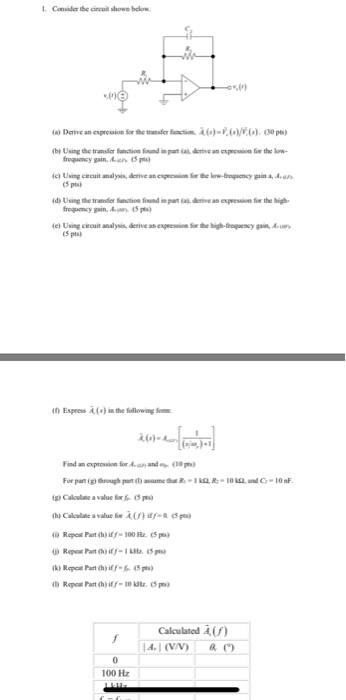L Consider the circuit shown below by Using the traar fanction found in pat (ak derive an cxpression for the ow c) Using circuit analysis, derive an epssio o te lorw-froquency gain a, Aun (5 pts (d) Using the transfer fatile fundi.~ส6aa.deme an epression fr the hi froguency gain, (5p) e) Using cecuit analysis, derive an expssion o te high-frogency ga,A (5 pts) Expeess A (s) in the following o Find an exprossion for Aan ande (30pes For parig,through h@aunetha...

• Consider the RLC circuit shown in the figure driven by a sinusoidal emf. Use the following...Consider the RLC circuit shown in the figure driven by a sinusoidal emf. Use the following data: ERMS= 145 V, R = 840 2, L = 37.0 mH, C = 0.150 mF. R ww Calculate the RMS current through the capacitor at the resonance frequency. Submit Answer Tries 0/12 What is the resonant frequency of this circuit? Submit Answer Tries 0/12 Calculate the RMS voltage across the inductor at the resonance frequency. Submit Answer Tries 0/12

• in the circuit below, R=50 ohms, L= 50 mH and C=680 microF determine ping Tool t...in the circuit below, R=50 ohms, L= 50 mH and C=680 microF determine ping Tool t Tools Help - Mode v Delay - HD SM S In the circuit below, R = 50 Ohms, L = 50 mH and C = 680 uF. Determine: a) The transfer function H(s) b) The resonant frequency in Hertz c) The bandwidth in Hertz d) The upper cutoff frequency in Hertz e) The lower cutoff frequency in Hertz f) Which type of filter is...

• QUESTION #2 PLEASE 1. Derive the transfer function for the circuit shown below. Plot H(s) versus...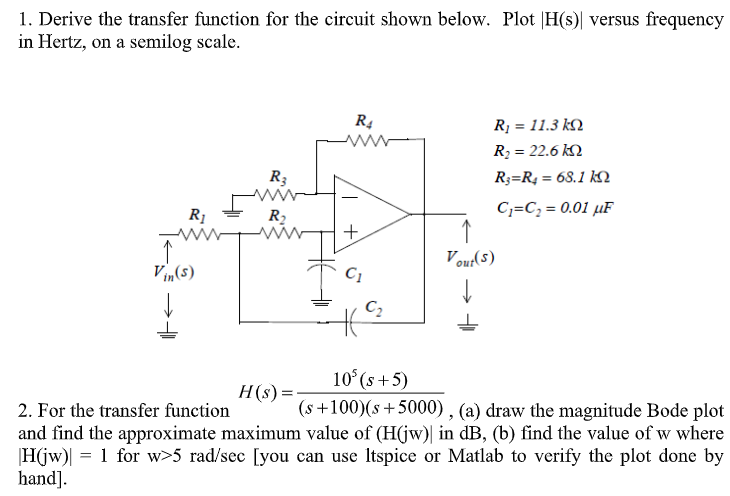QUESTION #2 PLEASE 1. Derive the transfer function for the circuit shown below. Plot H(s) versus frequency in Hertz, on a semilog scale. Ri 11.3 k Ri 22.6 k R R = 68.1 kN R3 C C 0.01 uF R2 Vout(s) Vin(s) C2 10 (s+5) H(s) = (s+100)(s5000) , (a) draw the magnitude Bode plot 2. For the transfer function and find the approximate maximum value of (H(jw) in dB, (b) find the value of w where 1 for w>5...